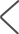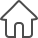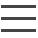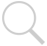已知抛物线y平方=2px(p＞0）过焦点斜率为2√2的直线交抛物线于A（x,y)B(x底数为二y底数为二）（X底数为一＜X底数为二）两点且|AB|=9 1）求抛物线的方程 2）0为坐标原点 C为抛物线上一点 若向量OC=向量OA+良母他向量OB求良母他的值×

9-99元的随机现金红包```已知抛物线y平方=2px(p＞0）过焦点斜率为2√2的直线交抛物线于A（x,y)B(x底数为二y底数为二）（X底数为一＜X底数为二）两点且|AB|=9
1）求抛物线的方程
2）0为坐标原点 C为抛物线上一点 若向量OC=向量OA+良母他向量OB求良母他的值```

```解：焦点F(p/2,0)，过焦点的直线方程为y=2√2(x-p/2)，代入抛物线方程y^2=2px并化简得
4x^2-5px+p^2=0    ①

x1+x2=5/4*p         ②
x1x2=1/4*p^2        ③

9=√[1+(2√2)^2]*√[(x1+x2)^2-4x1x2]=3*√[(5/4*p)^2-4*1/4*p^2]=3*3/4*p=9/4*p

A(1,-2√2),B(4,4√2).则直线OB的斜率为4√2/4=√2,于是直线AC的方程为y+2√2=√2(x-1)，即
y=√2(x-3)，代入y^2=8x，可得(x-1)(x-9)=0,解得x=1或x=9。显然x=1对应点A(1,-2√2),x=9对应点C(9,6√2)。于是λ=AC/OB=(6√2-1)/(4-0)=(6√2-1)/4  （因为斜率都是√2，故线段长度之比等于横坐标差之比）```